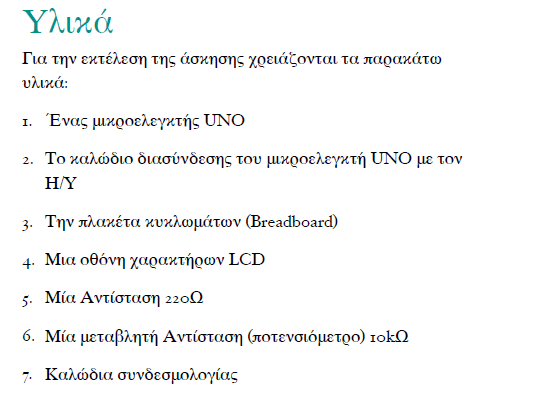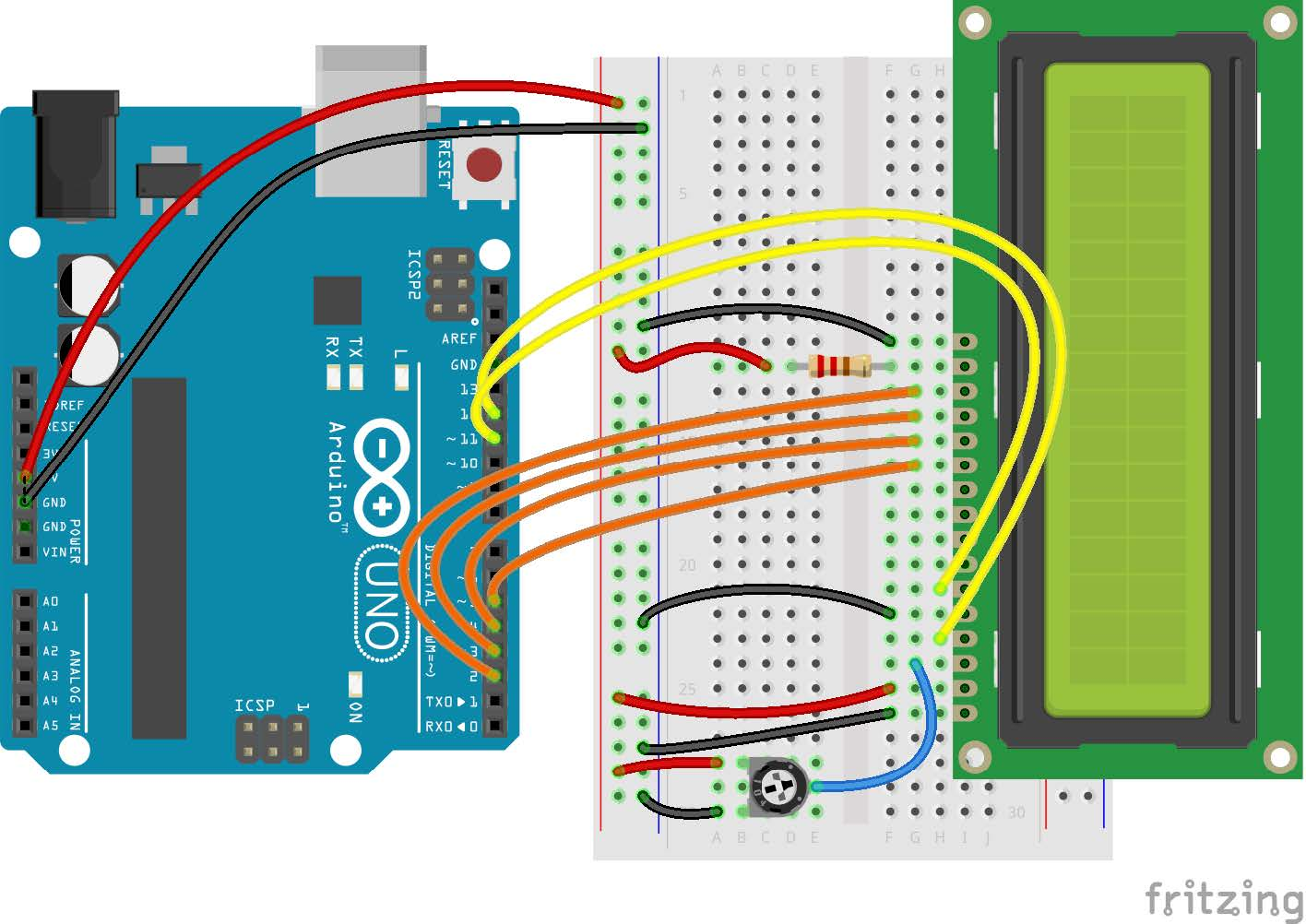tel. 24910 23755 / fax 24910 26620`/*`
`Display messages in an LCD display`
`*/`
`// include LCD library headers`
`#include <LiquidCrystal.h>`
`// setup LCD`
`// RS E D4 D5 D6 D7`
`LiquidCrystal lcd(12, 11, 5, 4, 3, 2);`
`// the setup routine runs once when you press`
`// reset:`
`void setup() {`
`// initialize the LCD`
`lcd.begin(16, 2);`
`// print message`
`lcd.print("hello, World!");`
`}`
`// the loop routine runs over and over again`
`// forever:`
`void loop() {`
`  // print the number of milliseconds since the`
`  // Arduino was reset`
`  lcd.setCursor(0, 1);`
`  lcd.print(millis()/1000);`
`}`

Κατηγορίες: Arduino